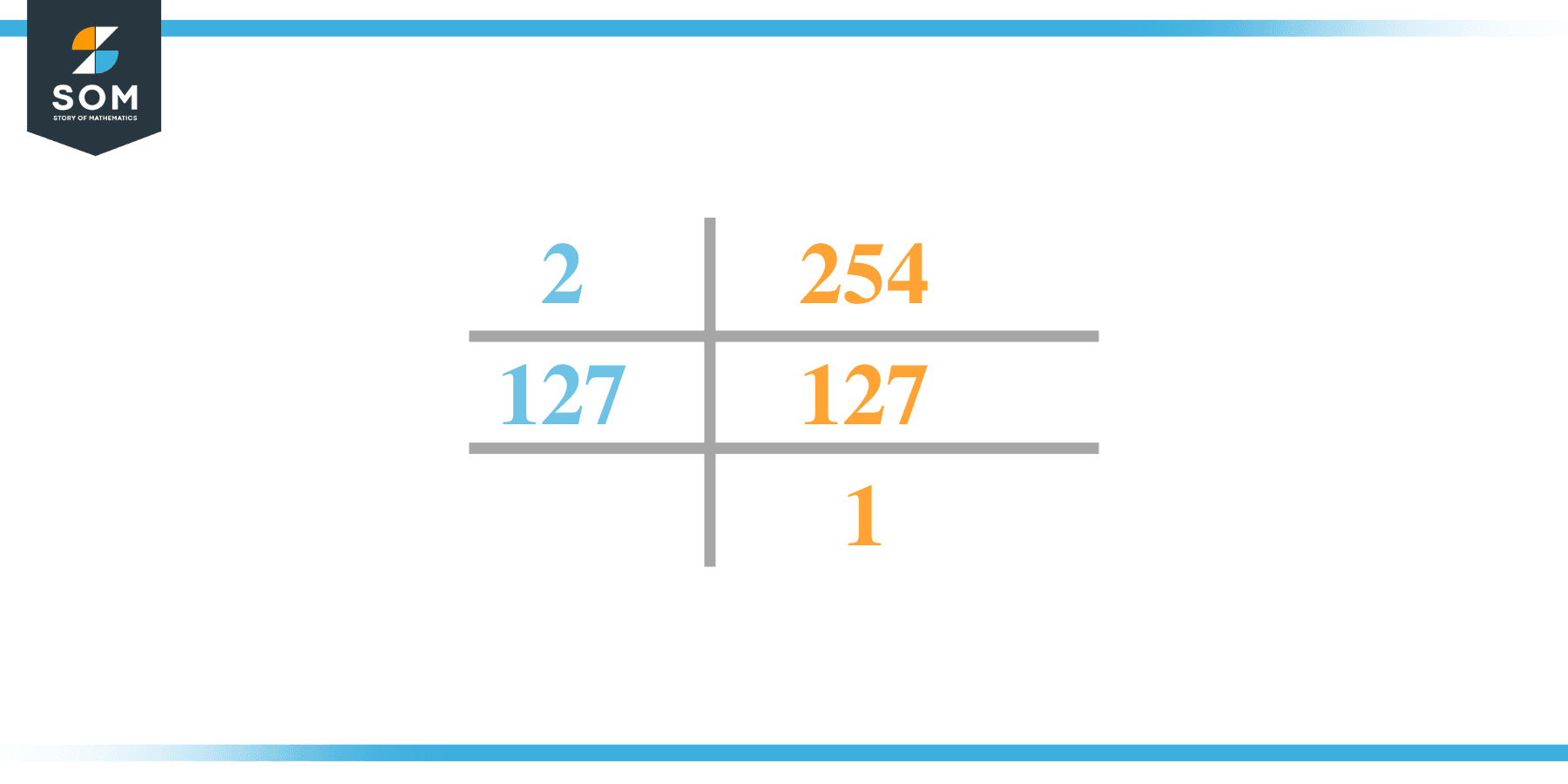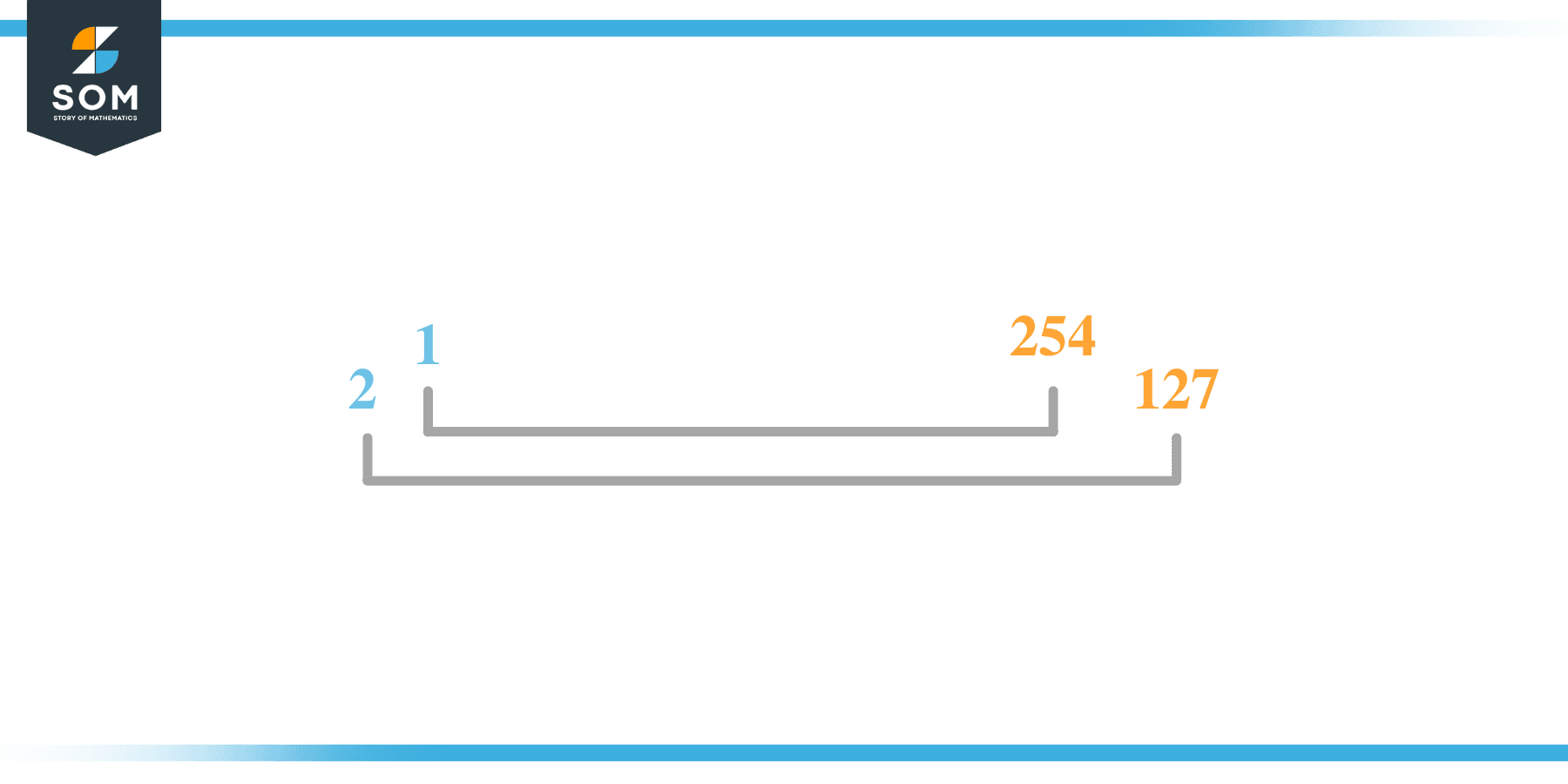# Factors of 254: Prime Factorization, Methods, and Examples

The factors of 254 are numbers that produce 254 as the product when multiplied together.The given number’s factors can be positive and negative, provided that the given number is achieved upon multiplication of two-factor integers.

### Factors of 254

Here are the factors of number 254.

Factors of 254: 1, 2, 127 and 254

### Negative Factors of 254

The negative factors of 254 are similar to its positive factors, just with a negative sign.

Negative Factors of 254: -1, -2, -127 and -254

### Prime Factorization of 254

The prime factorization of 254 is the way of expressing its prime factors in the product form.

Prime Factorization: 2 x 127

In this article, we will learn about the factors of 254 and how to find them using various techniques such as upside-down division, prime factorization, and factor tree.

## What Are the Factors of 254?

The factors 254 are 1, 2, 127, and 254. All of these numbers are the factors as they do not leave any remainder when divided by 254.

The factors of 254 are classified as prime numbers and composite numbers. The prime factors of the number 254 can be determined using the prime factorization technique.

## How To Find the Factors of 254?

You can find the factors of 254 by using the rules of divisibility. The divisibility rule states that any number, when divided by any other natural number, is said to be divisible by the number if the quotient is the whole number and the resulting remainder is zero.

To find the factors of 254, create a list containing the numbers divisible by 254 with zero remainders. One important thing to note is that 1 and 254 are the 254’s factors as every natural number has 1 and the number itself as its factor.

1 is also called the universal factor of every number. The factors of 254 are determined as follows:

$\dfrac{254}{1} = 254$

$\dfrac{254}{2} = 127$

$\dfrac{254}{127} = 2$

$\dfrac{254}{254} = 1$

Therefore, 1, 2, 127 and 254 are the factors of 254.

### Total Number of Factors of 254

For 254, there are four positive factors and four negative ones. So in total, there are eight factors of 254.

To find the total number of factors of the given number, follow the procedure mentioned below:

1. Find the factorization of the given number.
2. Demonstrate the prime factorization of the number in the form of exponent form.
3. Add 1 to each of the exponents of the prime factor.
4. Now, multiply the resulting exponents together. This obtained product is equivalent to the total number of factors of the given number.

By following this procedure, the total number of factors of 254 is given as:

Factorization of 254 is 1 x 2 x 127

The exponent of 1, 2, and 127 is 1.

Adding 1 to each and multiplying them together results in 8.

Therefore, the total number of factors of 254 is 8. 4 are positive, and four are negative.

### Important Notes

Here are some important points that must be considered while finding the factors of any given number:

• The factor of any given number must be a whole number.
• The factors of the number cannot be in the form of decimals or fractions.
• Factors can be positive as well as negative.
• Negative factors are the additive inverse of the positive factors of a given number.
• The factor of a number cannot be greater than that number.
• Every even number has 2 as its prime factor, the smallest prime factor.

## Factors of 254 by Prime FactorizationThe number 254 is a composite number. Prime factorization is a useful technique for finding the number’s prime factors and expressing the number as the product of its prime factors.

Before finding the factors of 254 using prime factorization, let us find out what prime factors are. Prime factors are the factors of any given number that are only divisible by 1 and themselves.

To start the prime factorization of 254, start dividing by its smallest prime factor. First, determine that the given number is either even or odd. If it is an even number, then 2 will be the smallest prime factor.

Continue splitting the quotient obtained until 1 is received as the quotient. The prime factorization of 254 can be expressed as:

254 = 2 x 127

## Factors of 254 in PairsThe factor pairs are the duplet of numbers that, when multiplied together, result in the factorized number. Factor pairs can be more than one depending on the total number of factors of the given numbers.

For 254, the factor pairs can be found as:

1 x 254 = 254

2 x 127 = 254

The possible factor pairs of 254 are given as (1, 254) and (2, 127).

All these numbers in pairs, when multiplied, give 254 as the product.

The negative factor pairs of 254are given as:

-1 x -254 = 254

-2 x -127 = 254

It is important to note that in negative factor pairs, the minus sign has been multiplied by the minus sign, due to which the resulting product is the original positive number. Therefore, -1, -2, -127, and -254 are called negative factors of 254.

The list of all the factors of 254, including positive and negative numbers, is given below.

Factor list of 254: 1, -1, 2, -2, 127, -127, 254 and -254

## Factors of 254 Solved Examples

To better understand the concept of factors, let’s solve some examples.

### Example 1

How many factors of 254 are there?

### Solution

The total number of Factors of 254 is 4.

Factors of 254 are 1, 2, 127, and 254.

### Example 2

Find the factors of 254 using prime factorization.

### Solution

The prime factorization of 254 is given as:

254 $\div$ 2 = 127

127 $\div$ 127 = 1

So the prime factorization of 254 can be written as:

2 x 127 = 254